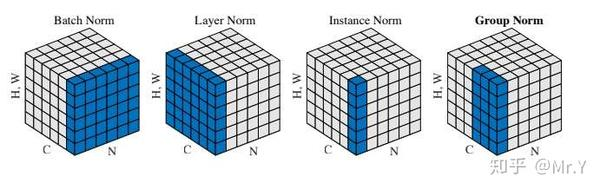# Normalization

## normalization¶

Batch Normalization (BN)
layer-normalization Layer Normalization (LN)
Instance Normalization (IN)
Group Normalization (GN)
weight-normalization
spectral-normalization

### tldr¶

bn

• bn对除channel外的所有维做归一化
• 得到C通道（类似特征）个均值和方差
• 推理时可以做参数融合加快推理速度
• 对 batch 大小敏感

ln

• ln对除batch外的所有维做归一化（nlp中只对channel做归一化，不同场景下不同，这个要注意！）
• 不受 batch size/句子长度影响，适用于RNN
• 如果不同输入特征不属于相似的类别（比如颜色和大小），那么 LN 的处理可能会降低模型的表达能力。
• 一个可信的直观解释：nlp中emmbedding并不存在一个客观的分布，相反我们需要考虑的是：我们希望得到一个符合什么样分布的embedding?
• 很好理解，通过layer normalization得到的embedding是 以坐标原点为中心，中空的球形环状分布，越往两侧越稀疏的环形球体空间中。

### summary¶

$y=\gamma(\frac{x-\mu(x)}{\sigma(x)})+\beta(1)$

### Batch Normalization¶

result: 得到 C 个均值，C 为通道数

nn.BatchNorm2d()

ps: 这里只是粗糙的公式，细节计算见： https://zhuanlan.zhihu.com/p/120265831

$\mu_{c}(x)=\frac{1}{N H W} \sum_{n=1}^{N} \sum_{h=1}^{H} \sum_{w=1}^{W} x_{n c h w}(2)$
$\sigma_{c}(x)=\sqrt{\frac{1}{N H W} \sum_{n=1}^{N} \sum_{h=1}^{H} \sum_{w=1}^{W}\left(x_{n c h w}-\mu_{c}(x)\right)^{2}+\epsilon}(4)$

# normalization
import torch
from torch import nn

# track_running_stats=False，求当前 batch 真实平均值和标准差，
# 而不是更新全局平均值和标准差
# affine=False, 只做归一化，不乘以 gamma 加 beta（通过训练才能确定）
# num_features 为 feature map 的 channel 数目
# eps 设为 0，让官方代码和我们自己的代码结果尽量接近
bn = nn.BatchNorm2d(num_features=3, eps=0, affine=False, track_running_stats=False)

# 乘 10000 为了扩大数值，如果出现不一致，差别更明显
x = torch.rand(10, 3, 5, 5)*10000
official_bn = bn(x)

# 把 channel 维度单独提出来，而把其它需要求均值和标准差的维度融合到一起
x1 = x.permute(1,0,2,3).view(3, -1)

mu = x1.mean(dim=1).view(1,3,1,1)
# unbiased=False, 求方差时不做无偏估计（除以 N-1 而不是 N），和原始论文一致
# 个人感觉无偏估计仅仅是数学上好看，实际应用中差别不大
std = x1.std(dim=1, unbiased=False).view(1,3,1,1)

my_bn = (x-mu)/std

diff=(official_bn-my_bn).sum()
print('diff={}'.format(diff)) # 差别是 10-5 级的，证明和官方版本基本一致


### 为什么会带来随机性¶

Batch normalization 的主要目的是减少神经网络中深层层次之间的共变量转移，以及减轻梯度消失和梯度爆炸的问题。在进行训练时，batch normalization 除了对每个 mini-batch 进行归一化之外，还会进行平移和缩放操作。这些参数平移和缩放操作会引入额外的随机性，因为每个 mini-batch 的统计信息都不同, 与之对比的是采用全局平均值和方差。

### 滑动平均¶

$$\mu_{i}=\theta\mu_{i-1} + (1-\theta)\mu_{i}$$

5%提速 + 节省内存

## Layer Normalization¶

result:
CV: 中得到 N 个均值， N 为样本/batch_size个数，对 C + H + W 上做归一化
NLP: 中得到(batch, seq_size)个，相当于对 emb或者hidden_size 上做归一化

BN 的一个缺点是需要较大的 batchsize 才能合理估训练数据的均值和方差，这导致内存很可能不够用，同时它也很难应用在训练数据长度不同的 RNN 模型上。Layer Normalization (LN) 的一个优势是不需要批训练，在单条数据内部就能归一化。

$\mu_{n}(x)=\frac{1}{C H W} \sum_{c=1}^{C} \sum_{h=1}^{H} \sum_{w=1}^{W} x_{n c h w}$
$\sigma_{n}(x)=\sqrt{\frac{1}{C H W} \sum_{c=1}^{C} \sum_{h=1}^{H} \sum_{w=1}^{W}\left(x_{n c h w}-\mu_{n}(x)\right)^{2}+\epsilon}$
class LayerNorm(nn.Module):
def __init__(self, d_model, eps=1e-12):
super(LayerNorm, self).__init__()
self.gamma = nn.Parameter(torch.ones(d_model))
self.beta = nn.Parameter(torch.zeros(d_model))
self.eps = eps

def forward(self, x):
mean = x.mean(-1, keepdim=True)
var = x.var(-1, unbiased=False, keepdim=True)
# '-1' means last dimension.

out = (x - mean) / torch.sqrt(var + self.eps)
out = self.gamma * out + self.beta
return out


• batch_size：一次训练或推断时输入数据的样本数。
• sequence_length：输入序列的长度，对于Transformer来说就是指单词序列的长度。
• hidden_size：隐藏层的大小，即向量的维度。

Layer normalization是对隐藏状态向量的最后一个维度（hidden_size）进行归一化的。

import torch
from torch import nn

x = torch.rand(10, 3, 5, 5)*10000

# normalization_shape 相当于告诉程序这本书有多少页，每页多少行多少列
# eps=0 排除干扰
# elementwise_affine=False 不作映射
# 这里的映射和 BN 以及下文的 IN 有区别，它是 elementwise 的 affine，
# 即 gamma 和 beta 不是 channel 维的向量，而是维度等于 normalized_shape 的矩阵
ln = nn.LayerNorm(normalized_shape=[3, 5, 5], eps=0, elementwise_affine=False)

official_ln = ln(x)

x1 = x.view(10, -1)
mu = x1.mean(dim=1).view(10, 1, 1, 1)
std = x1.std(dim=1,unbiased=False).view(10, 1, 1, 1)

my_ln = (x-mu)/std

diff = (my_ln-official_ln).sum()

print('diff={}'.format(diff)) # 差别和官方版本数量级在 1e-5


## Instance Normalization¶

IN一般用于生成任务和风格迁移任务，因为这种任务会对细节特征有高要求，直观可以理解为更细粒度的特征区分要求

Instance Normalization (IN) 最初用于图像的风格迁移。作者发现，在生成模型中， feature map 的各个 channel 的均值和方差会影响到最终生成图像的风格，因此可以先把图像在 channel 层面归一化，然后再用目标风格图片对应 channel 的均值和标准差“去归一化”，以期获得目标图片的风格。IN 操作也在单个样本内部进行，不依赖 batch。

$\mu_{n c}(x)=\frac{1}{H W} \sum_{h=1}^{H} \sum_{w=1}^{W} x_{n c h w}(6)$
$\sigma_{n c}(x)=\sqrt{\frac{1}{H W} \sum_{h=1}^{H} \sum_{w=1}^{W}\left(x_{n c h w}-\mu_{n c}(x)\right)^{2}+\epsilon}$

IN 求均值时，相当于把一页书中所有字加起来，再除以该页的总字数：H×W，即求每页书的“平均字”，求标准差时也是同理。

import torch
from torch import nn

x = torch.rand(10, 3, 5, 5) * 10000

# track_running_stats=False，求当前 batch 真实平均值和标准差，
# 而不是更新全局平均值和标准差
# affine=False, 只做归一化，不乘以 gamma 加 beta（通过训练才能确定）
# num_features 为 feature map 的 channel 数目
# eps 设为 0，让官方代码和我们自己的代码结果尽量接近
In = nn.InstanceNorm2d(num_features=3, eps=0, affine=False, track_running_stats=False)

official_in = In(x)

x1 = x.view(30, -1)
mu = x1.mean(dim=1).view(10, 3, 1, 1)
std = x1.std(dim=1, unbiased=False).view(10, 3, 1, 1)

my_in = (x-mu)/std

diff = (my_in-official_in).sum()
print('diff={}'.format(diff)) # 误差量级在 1e-5


Instance Normalization: The Missing Ingredient for Fast Stylization <https://arxiv.org/abs/1607.08022>__.

$$y = \frac{x - \mathrm{E}[x]}{ \sqrt{\mathrm{Var}[x] + \epsilon}} * \gamma + \beta$$

## Group Normalization¶

Group Normalization (GN) 适用于占用显存比较大的任务，例如图像分割。对这类任务，可能 batchsize 只能是个位数，再大显存就不够用了。而当 batchsize 是个位数时，BN 的表现很差，因为没办法通过几个样本的数据量，来近似总体的均值和标准差。GN 也是独立于 batch 的，它是 LN(G=C) 和 IN(G=1)的折中, 。正如提出该算法的论文展示的：GN 计算均值和标准差时，把每一个样本 feature map 的 channel 分成 G 组，每组将有 C/G 个 channel，然后将这些 channel 中的元素求均值和标准差。各组 channel 用其对应的归一化参数独立地归一化。

$\mu_{n g}(x)=\frac{1}{(C / G) H W} \sum_{c=g C / G}^{(g+1) C / G} \sum_{h=1}^{H} \sum_{w=1}^{W} x_{n c h w}(8)$
$\sigma_{n g}(x)=\sqrt{\frac{1}{(C / G) H W} \sum_{c=g C / G}^{(g+1) C / G} \sum_{h=1}^{H} \sum_{w=1}^{W}\left(x_{n c h w}-\mu_{n g}(x)\right)^{2}+\epsilon}(9)$

import torch
from torch import nn

x = torch.rand(10, 20, 5, 5)*10000

# 分成 4 个 group
# 其余设定和之前相同
gn = nn.GroupNorm(num_groups=4, num_channels=20, eps=0, affine=False)
official_gn = gn(x)

# 把同一 group 的元素融合到一起
x1 = x.view(10, 4, -1)
mu = x1.mean(dim=-1).reshape(10, 4, -1)
std = x1.std(dim=-1).reshape(10, 4, -1)

x1_norm = (x1-mu)/std
my_gn = x1_norm.reshape(10, 20, 5, 5)

diff = (my_gn-official_gn).sum()

print('diff={}'.format(diff)) # 误差在 1e-4 级


## weight normalization WN¶

Weight Normalization

$$y=\phi(\boldsymbol{wx}+b)$$

WN的重参数表示：对权重w \bm{w}w用参数向量v和标量g进行表示，则新参数表示为：
$$\(\boldsymbol{w}=\frac{g}{\|\boldsymbol{v}\|}\boldsymbol{v}$$\)

WN的做法是将权值向量 $$W$$

weight_g、weight_v都会进行更新，各自独立更新，文中解释了，这样更新比单独更新W会更加稳定训练。

WN也是和样本量无关的，所以可以应用在batchsize较小以及RNN等动态网络中；另外BN使用的基于mini-batch的归一化统计量代替全局统计量，相当于在梯度计算中引入了噪声。而WN则没有这个问题，所以在生成模型，强化学习等噪声敏感的环境中WN的效果也要优于BN。

WN没有一如额外参数，这样更节约显存。同时WN的计算效率也要优于要计算归

hifigan 中用到！

from torch.nn.utils import weight_norm, remove_weight_norm, spectral_norm


## Spectral Normalization 谱归一化¶

### tutorial¶

https://zhuanlan.zhihu.com/p/63957812

### note¶

Spectral normalization for generative adversarial network” (以下简称 Spectral Norm) 使用一种更优雅的方式使得判别器 D 满足利普希茨连续性，限制了函数变化的剧烈程度，从而使模型更稳定。

## 总结¶

BN 相当于把这些书按页码一一对应地加起来（例如：第1本书第36页，加第2本书第36页……），再除以每个页码下的字符总数：N×H×W，因此可以把 BN 看成求“平均书”的操作（注意这个“平均书”每页只有一个字）

LN 相当于把每一本书的所有字加起来，再除以这本书的字符总数：C×H×W，即求整本书的“平均字”

IN 相当于把一页书中所有字加起来，再除以该页的总字数：H×W，即求每页书的“平均字”

GN 相当于把一本 C 页的书平均分成 G 份，每份成为有 C/G 页的小册子，对这个 C/G 页的小册子，求每个小册子的“平均字”

Conditional Batch Normalization详解

https://zhuanlan.zhihu.com/p/61248211

https://zhuanlan.zhihu.com/p/57875010

### nn.instanceNorm2d¶

bn对除channel外的所有维做归一化，ln对除batch外的所有维做归一化

Last update : 2023年12月4日
Created : 2023年8月4日